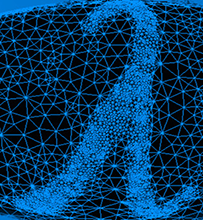# Spectral Graph TheoryLecture 1: Introduction to Spectral Graph Theory
Lecture 2: Expanders and Eigenvalues
Lecture 3: Small-set Expanders, Clustering, and Eigenvalues

#### This series of talks was part of the Algorithmic Spectral Graph Theory Boot Camp. Videos for each talk are available through the links above.

Speaker: Luca Trevisan, UC Berkeley

Spectral graph theory studies connections between combinatorial properties of graphs and the eigenvalues of matrices associated to the graph, such as the adjacency matrix and the Laplacian matrix.

Spectral graph theory has applications to the design and analysis of approximation algorithms for graph partitioning problems, to the study of random walks in graph, and to the construction of expander graphs. It also reveals connections between the above topics, and provides, for example, a way to use random walks to approximately solve graph partitioning problems.

In this series of lectures we will introduce the basics of spectral graph theory, we will prove the discrete Cheeger inequalities, and see them as (i) a way to approximate the sparsest cut problem, (ii) an approach to bound the mixing time of random walks, and (iii) a way to certify expansion in graphs. We will then consider various generalizations of this result, such as higher-order Cheeger inequalities, a Cheeger-like inequality for the largest eigenvalue of the Laplacian, and the expander mixing lemma and its inverse. Each of these more advanced results will also be interpreted as the analysis of an approximation algorithm for a graph partitioning problem.